# Some More Written Practice Questions

Question.1

A concave lens has focal length of 20 cm. At what distance from the lens a 5 cm tall object be placed so that it forms an image at 15 cm from the lens? Also calculate the size of the image formed. [Delhi]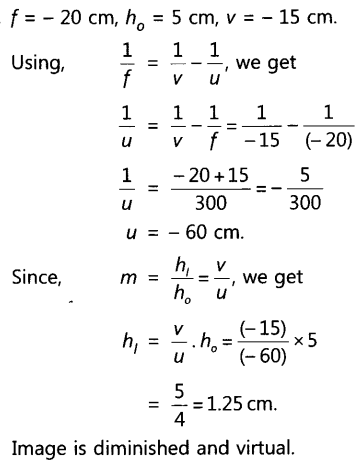Question.2

Draw the ray diagram in each case to show the position and nature of the image formed when the object is placed:
(i) at the centre of curvature of a concave mirror
(ii) between the pole P and focus F of a concave mirror
(iii) in front of a convex mirror
(iv) at 2F of a convex lens
(v) in front of a concave lens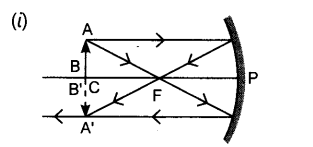Nature of image: Real, inverted and same size image is formed at the centre of curvature.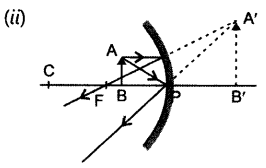Nature of image: Virtual, enlarged and erect image is formed behind the mirror.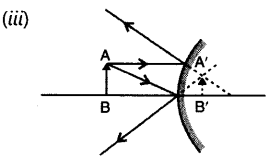Nature of image: Virtual, erect and diminished, image is formed behind the mirror.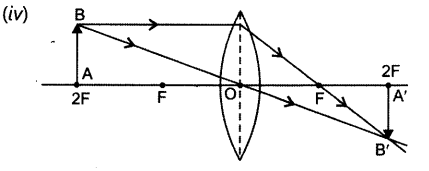Nature of image: Real, inverted and size to size, image is formed at 2F on the other side of lens.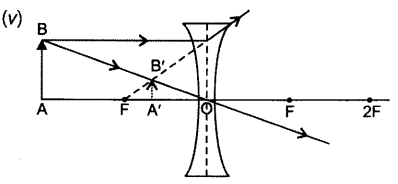Nature of image: Virtual, erect and diminished image is formed between O and F on the same side of object.

Question.3

The refractive indices of four media A, B, C and D are given in the following table:

If light, travels from one medium to another, in which case the change in speed will be (i) minimum, (ii) maximum? [Delhi(C)]

(i) Minimum change is seen as light moves between 1.50 and 1.52, i.e. B and C.
(ii) Maximum change when light moves between 1.33 and 2.40, i.e. A and D.

Question.4

List the sign conventions for reflection of light by spherical mirrors. Draw a diagram and apply these conventions in the determination of focal length of a spherical mirror which forms a three times magnified real image of an object placed 16 cm in front of it. [Delhi ]

Answer.  Sign conventions for reflection of light by spherical mirror are:

1.  The object is always placed to the left of the mirror.
2. All the distances parallel to the principal axis are always measured from the pole of the spherical mirror.
3.  All the distances measured along the direction of incident light (along +ve x-axis), are considered to be positive.
4. Those distances measured opposite to the direction of incidence light (i.e. along -ve x-axis), are taken as negative.
5. The distances measured in upward direction, i.e. perpendicular to and above the principal axis (along +ve y-axis), are taken as positive.
6. The distances measured in the downward direction, (along -ve y-axis), i.e. perpendicular to and below the principal axis are taken as negative.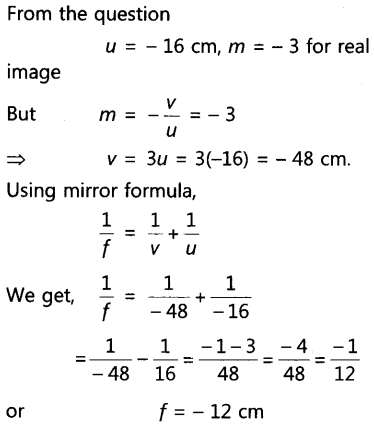So, focal length of spherical mirror is 12 cm. Negative sign of focal length indicates that mirror is concave in nature.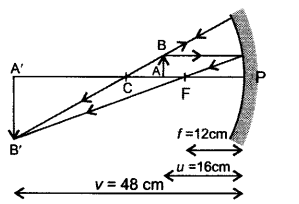Question.5

To construct a ray diagram, we use two light rays which are so chosen that it is easy to know their directions after reflection from the mirror. List these two rays and state the path of these rays after reflection. Use these rays to locate the image of an object placed between centre of curvature and focus of a concave mirror. [All India]